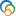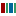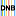The Library of Congress > Linked Data Service > LC Subject Headings (LCSH)

# Abel integral equations

• ### Variants

• Abel's integral equations
• Integral equations, Abel

• ### Closely Matching Concepts from Other Schemes

•Abel integral equations•Abel transformLabel from public data source Wikidata
•Abelsche Integralgleichung• ### Sources

• found: Work cat.: Gorenflo, R. Abel integral equations, 1991.
• found: Math. subj. classif.(Integral equations, 45-XX; Singular integral equations, 45Exx; Integral equations of the convolution type (Abel, Picard ...))
• found: Encyc. dict. math.:p. 830 (Abel's integral equation; a Volterra integral equation of the first kind)
• found: Encyc. math.
• notfound: LC data base, 1/9/91;PREMARC, 1/9/91

• QA431
• ### Change Notes

• 1991-01-14: new
• 1991-02-26: revised

# Suggest terminology

The LC Linked Data Service welcomes any suggestions you might have about terminology used for a given heading or concept.

Would you like to suggest a change to this heading?

Please provide your name, email, and your suggestion so that we can begin assessing any terminology changes.

Fields denoted with an asterisk (*) are required.